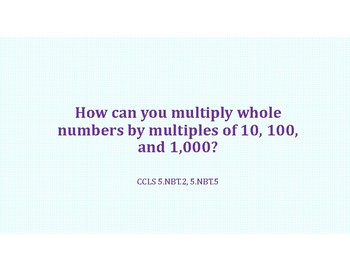# Unit 2 Multiply a Divide Whole Numbers and Decimals PowerPoints BundleSubject
Resource Type
File Type

PDF

(9 MB|274 pages)
Product Description
The PowerPoints typically include: Tiles/Links to Interactive Videos, Problem of the Day, Guided Practice, Independent Practice, and Agree or Disagree problems.

You can enjoy this bundle of all the Unit 2 PowerPoints for 20% off the price of all the PowerPoints separately! You can also purchase any of the other lesson's PowerPoints for Unit 2 separately in my store!

Unit 2 Multiply and Divide Whole Numbers and Decimals
-Lesson 1 Multiply whole numbers by multiples of ten
-Lesson 2 Multiply whole numbers by powers of ten
-Lesson 3 Multiply 2 digit by 2 digit numbers
-Lesson 4 Multiply 3 digit by 2 digit numbers
-Lesson 5 Multiply 4 digit by 2 digit whole numbers
-Lesson 6 Multiply decimals by powers of ten
-Lesson 7 Multiply a decimal by whole number
-Lesson 8 Multiply a decimal by a decimal
-Lesson 9 Divide a whole number by multiple of 10, 100, or 1000
-Lesson 10 Divide multi digit number by 1 digit number
-Lesson 11 Divide multi digit number by a 2 digit number
-Lesson 12 Divide a multi digit number with a remainder
-Lesson 13 Divide a decimal by power of ten
-Lesson 14 Divide a decimal by a whole number
-Lesson 15 Divide a decimal by a decimal
-Lesson 16 Order of operations
-Lesson 17 Order of operations with grouping symbols
-Lesson 18 Numerical Expressions
Total Pages
274 pages
N/A
Teaching Duration
N/A
Report this Resource to TpT
Reported resources will be reviewed by our team. Report this resource to let us know if this resource violates TpT’s content guidelines.